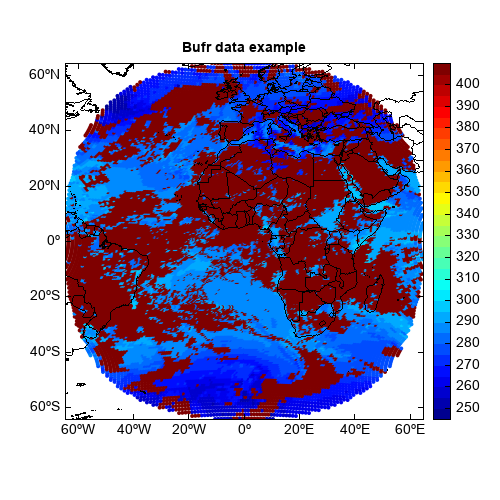BURF data file can be opened using `addfile()` function. Only a `obs` variable with `sequence` data type exists in the file. The members can be got using `get_members` method the the variable. Then the member array can be read using `member_array` method.

`keepopen=True` argument should be added in `addfile()` function so the data could be read from the opened file. The file object should be closed after data reading.

```>>> v.get_members()
[SATELLITE_IDENTIFIER, IDENTIFICATION_OF_ORIGINATING_GENERATING_CENTRE, SATELLITE_CLASSIFICATION, SEGMENT_SIZE_AT_NADIR_IN_X-DIRECTION, SEGMENT_SIZE_AT_NADIR_IN_Y-DIRECTION, YEAR, MONTH, DAY, HOUR, MINUTE, SECOND, ROW_NUMBER, COLUMN_NUMBER, LATITUDE_(HIGH_ACCURACY), LONGITUDE_(HIGH_ACCURACY), CLOUD_AMOUNT_IN_SEGMENT, AMOUNT_OF_LOW_CLOUDS, NUMBER_OF_OBSERVATIONS, struct1, AMOUNT_OF_MIDDLE_CLOUDS, NUMBER_OF_OBSERVATIONS-2, struct2, AMOUNT_OF_HIGH_CLOUDS, NUMBER_OF_OBSERVATIONS-3, struct3, DATA_PRESENT_INDICATOR, IDENTIFICATION_OF_ORIGINATING_GENERATING_CENTRE-2, GENERATING_APPLICATION, OBSERVATION_QUALITY]
```

Example script

```fn = 'D:/Temp/bufr/MSG3-SEVI-MSGCLAP-0000-0000-20150101004500.000000000Z-20150101005935-1187380.bfr'
v = f['obs']
lon = v.member_array('LONGITUDE_(HIGH_ACCURACY)')
lat = v.member_array('LATITUDE_(HIGH_ACCURACY)')
lon = (lon - 1.8E7) * 1.E-5
lat = (lat - 9.E6) * 1.E-5
struct1 = v.member_array('struct1')
temp = struct1.member_array('TEMPERATURE_AIR_TEMPERATURE')
temp = temp * 0.1
temp = temp[:,0]
f.close()

#Plot
axesm()
geoshow('country')
layer = scatter(lon, lat, temp, 20, size=4, edge=False, zorder=0)
colorbar(layer)
title('Bufr data example')
```